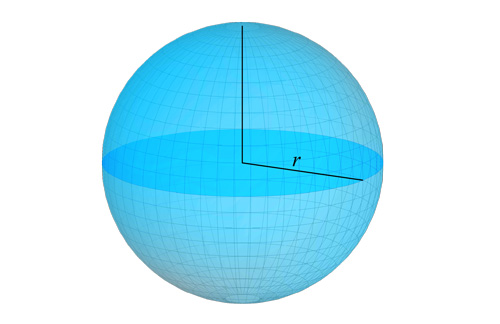The ball is one of the fundamental geometric shapes. In real life, balls of perfect shape are not found very often, but at school or university you may encounter the task of calculating the volume of the ball. Either you need to make balls for the bearing or calculate the volume of basketballs. We suggest you use our simple and quick ball volume calculator. You can also convert the result to the desired measure of volume.The radius of a ball is the distance from its center to its edge

## Ball volume formula

The volume of the ball is calculated by the formula V = 4 / 3πr³ .

This formula is the same in the whole world. It is as accurate as possible with respect to balls of perfect shape, but you can calculate the approximate volume of imperfect earth objects.Let your balls always stay in perfect shape